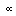# U+221D PROPORTIONAL TO

U+221D was added to Unicode in version 1.1 (1993). It belongs to the blockMathematical Operators in the Basic Multilingual Plane.

This character is a Math Symbol and is commonly used, that is, in no specific script.

The glyph is not a composition. It has a Ambiguous East Asian Width. In bidirectional context it acts as Other Neutral and is mirrored. In text U+221D behaves as Ambiguous regarding line breaks. It has type Other for sentence and Other for word breaks. The Grapheme Cluster Break is Any.

In mathematics, two variables are proportional if a change in one is always accompanied by a change in the other, and if the changes are always related by use of a constant multiplier. The constant is called the coefficient of proportionality or proportionality constant.

• If one variable is always the product of the other and a constant, the two are said to be directly proportional. x and y are directly proportional if the ratio is constant.
• If the product of the two variables is always equal to a constant, the two are said to be inversely proportional. x and y are inversely proportional if the product xy is constant.

In order to express the statement "y is proportional to x" mathematically, we write an equation y = cx, for some real constant, c. Symbolically, this is written yx.

In order to express the statement "y is inversely proportional to x" mathematically, we write an equation y = c/x. We can equivalently write "y is proportional to 1/x", which y = c/x would represent.

If a linear function transforms 0, a and b into 0, c and d, and if the product a b c d is not zero, we say a and b are proportional to c and d. An equality of two ratios such as , where no term is zero, is called a proportion.

## Representations

System Representation
8733
UTF-8 E2 88 9D
UTF-16 22 1D
UTF-32 00 00 22 1D
URL-Quoted %E2%88%9D
HTML-Escape &#x221D;
Wrong windows-1252 Mojibake â
HTML-Escape &prop;
HTML-Escape &Proportional;
HTML-Escape &propto;
HTML-Escape &varpropto;
HTML-Escape &vprop;
digraph 0(
LaTeX \propto
Encoding: EUC-KR (hex bytes) A1 F0
Encoding: JIS0208 (hex bytes) A2 E7

## Complete Record

Property Value
Age (age) 1.1
Unicode Name (na) PROPORTIONAL TO
Unicode 1 Name (na1)
Block (blk) Math_Operators
General Category (gc) Math Symbol
Script (sc) Common
Bidirectional Category (bc) Other Neutral
Combining Class (ccc) Not Reordered
Decomposition Type (dt) None
Decomposition Mapping (dm)Lowercase (Lower)
Simple Lowercase Mapping (slc)Lowercase Mapping (lc)Uppercase (Upper)
Simple Uppercase Mapping (suc)Uppercase Mapping (uc)Simple Titlecase Mapping (stc)Titlecase Mapping (tc)Case Folding (cf)ASCII Hex Digit (AHex)
Alphabetic (Alpha)
Bidi Control (Bidi_C)
Bidi Mirrored (Bidi_M)
Bidi Paired Bracket (bpb)Bidi Paired Bracket Type (bpt) None
Cased (Cased)
Composition Exclusion (CE)
Case Ignorable (CI)
Full Composition Exclusion (Comp_Ex)
Changes When Casefolded (CWCF)
Changes When Casemapped (CWCM)
Changes When NFKC Casefolded (CWKCF)
Changes When Lowercased (CWL)
Changes When Titlecased (CWT)
Changes When Uppercased (CWU)
Dash (Dash)
Deprecated (Dep)
Default Ignorable Code Point (DI)
Diacritic (Dia)
East Asian Width (ea) Ambiguous
Extender (Ext)
FC NFKC Closure (FC_NFKC)Grapheme Cluster Break (GCB) Any
Grapheme Base (Gr_Base)
Grapheme Extend (Gr_Ext)
Hex Digit (Hex)
Hangul Syllable Type (hst) Not Applicable
Hyphen (Hyphen)
ID Continue (IDC)
Ideographic (Ideo)
ID Start (IDS)
IDS Binary Operator (IDSB)
IDS Trinary Operator and (IDST)
InMC (InMC)
Indic Positional Category (InPC) NA
Indic Syllabic Category (InSC) Other
ISO 10646 Comment (isc)
Joining Group (jg) No_Joining_Group
Join Control (Join_C)
Jamo Short Name (JSN)
Joining Type (jt) Non Joining
Line Break (lb) Ambiguous
Logical Order Exception (LOE)
Math (Math)
Noncharacter Code Point (NChar)
NFC Quick Check (NFC_QC) Yes
NFD Quick Check (NFD_QC) Yes
NFKC Casefold (NFKC_CF)NFKC Quick Check (NFKC_QC) Yes
NFKD Quick Check (NFKD_QC) Yes
Numeric Type (nt) None
Numeric Value (nv) NaN
Other Alphabetic (OAlpha)
Other Default Ignorable Code Point (ODI)
Other Grapheme Extend (OGr_Ext)
Other ID Continue (OIDC)
Other ID Start (OIDS)
Other Lowercase (OLower)
Other Math (OMath)
Other Uppercase (OUpper)
Pattern Syntax (Pat_Syn)
Pattern White Space (Pat_WS)
Quotation Mark (QMark)
Sentence Break (SB) Other
Simple Case Folding (scf)Script Extension (scx) Common
Soft Dotted (SD)
STerm (STerm)
Terminal Punctuation (Term)
Unified Ideograph (UIdeo)
Variation Selector (VS)
Word Break (WB) Other
White Space (WSpace)
XID Continue (XIDC)
XID Start (XIDS)
Expands On NFC (XO_NFC)
Expands On NFD (XO_NFD)
Expands On NFKC (XO_NFKC)
Expands On NFKD (XO_NFKD)